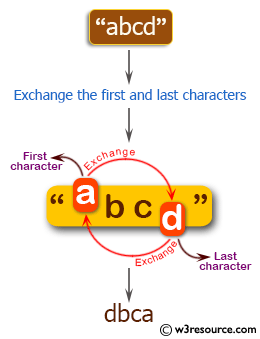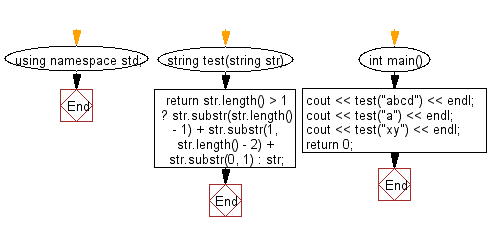﻿ C++ : Reverse the first and last elements of a string# C++ Exercises: Exchange the first and last characters in a given string and return the new string

## C++ Basic Algorithm: Exercise-7 with Solution

Write a C++ program to exchange the first and last characters in a given string and return the new string.

Sample Solution:

C++ Code :

``````#include <iostream>
using namespace std;

string test(string str)
{
return str.length() > 1
? str.substr(str.length() - 1) + str.substr(1, str.length() - 2) + str.substr(0, 1) : str;
}

int main()
{
cout << test("abcd") << endl;
cout << test("a") << endl;
cout << test("xy") << endl;
return 0;
}
``````

Sample Output:

```dbca
a
yx
```

Pictorial Presentation:Flowchart:C++ Code Editor: